# Pythagorean theorem - 7th grade (12y) - math problems

#### Number of problems found: 99

• Broken treeThe tree is broken at 4 meters above the ground and the top of the tree touches the ground at a distance of 5 from the trunk. Calculate the original height of the tree.
• Acreage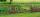What acreage has a rectangular plot whose diagonal is 34 meters long and one side has a length of 16 meters. ?
• OPT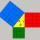What is the perimeter of a right triangle with the legs 14 cm and 21 cm long?Ladder 10 meters long is staying against the wall so that its bottom edge is 6 meters away from the wall. What height reaches ladder?
• Perimeter of circleCalculate the circumference of described circle to the triangle with sides 9,12,15 cm.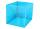I have homework. The cube has an edge 7 cm long and I must find wall and body diagonal.
• SquareRectangular square has side lengths 183 and 244 meters. How many meters will measure the path that leads straight diagonally from one corner to the other?Ladder 8 m long is leaning against the wall. Its foot is 1 m away from the wall. At which height ladder touches the wall?
• ArcCalculate span of the arc, which is part of a circle with diameter d = 20 m and its height is 6 m.
• Diameter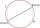If the endpoints of a diameter of a circle are A(10, -1) and B (3, 10), what is the radius of the circle?
• CirclesArea of circle inscribed in a square is 14. What is the area of a circle circumscribed around a square?
• RailwaysRailways climb 7.4 ‰. Calculate the height difference between two points on the railway distant 3539 meters.
• HypotenuseCalculate the length of the hypotenuse of a right triangle with a catheti 71 cm and 49 cm long.
• Rhombus 2Calculate the rhombus area, which has a height v=48 mm and shorter diagonal u = 60 mm long.
• Square s3Calculate the diagonal of the square, where its area is 0.49 cm square. And also calculate its circumference.
• Drainage channel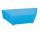The cross section of the drainage channel is an isosceles trapezoid whose bases have a length of 1.80 m, 0.90 m and arm has length 0.60 meters. Calculate the depth of the channel.
• Diagonals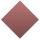Rhombus has two diagonals e=14 dm and f=11 dm. Calculate the side angle and height of the rhombus.
• MinuteTwo boys started from one place. First went north at velocity 3 m/s and the second to the east with velocity 4 m/s. How far apart they are after minute?
• Circumscribed circle to squareFind the length of a circle circumscribing a square of side 10 cm. Compare it to the perimeter of this square.
• Diagonal - simple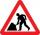Calculate the length of the diagonal of a rectangle with dimensions 5 cm and 12 cm.

Do you have an interesting mathematical word problem that you can't solve it? Submit a math problem, and we can try to solve it.

We will send a solution to your e-mail address. Solved examples are also published here. Please enter the e-mail correctly and check whether you don't have a full mailbox.

Please do not submit problems from current active competitions such as Mathematical Olympiad, correspondence seminars etc...

Pythagorean theorem is the base for the right triangle calculator. Pythagorean theorem - math word problems. Examples for 7th grade (seventh).